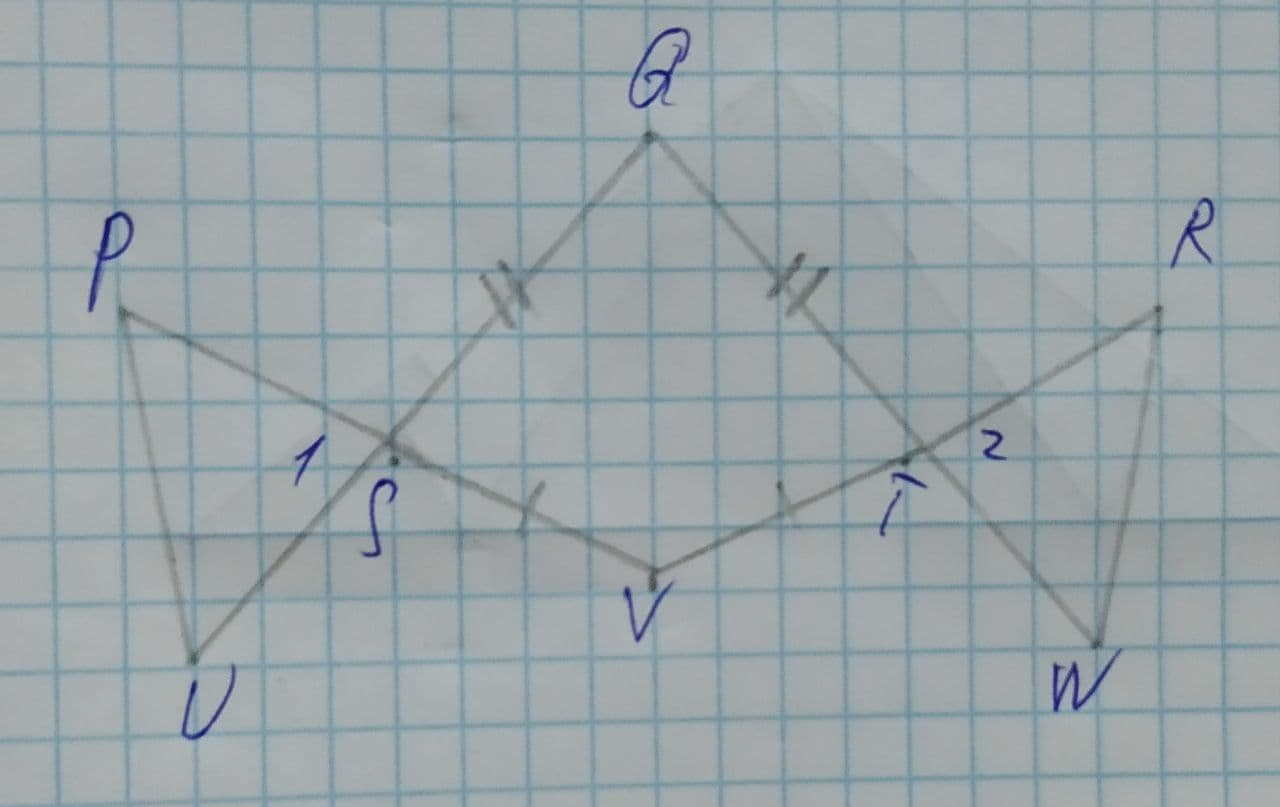Question# To write: The plan to prove \angle1\cong\angle2, use the given figure is as follows

Congruence
ANSWEREDTo write: The plan to prove $$\displaystyle\angle{1}\stackrel{\sim}{=}\angle{2}$$.
Given:
The given figure is as follows.By SSS Congruence Theorem, prove that $$\displaystyle\triangle{Q}{S}{V}\stackrel{\sim}{=}\triangle{Q}{T}{V}$$.
Then, state that $$\displaystyle\angle{Q}{S}{V}\stackrel{\sim}{=}\angle{Q}{T}{V}$$.
Use the Vertical Angles Theorem, $$\displaystyle\angle{Q}{S}{V}\stackrel{\sim}{=}\angle{1},\ {\quad\text{and}\quad}\ \angle{Q}{T}{V}\stackrel{\sim}{=}\angle{2}$$.
Therefore, by the Transitive Property of Congruence to prove that $$\displaystyle\angle{1}\stackrel{\sim}{=}\angle{2}$$.